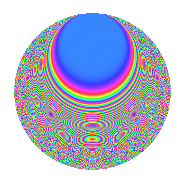Properties

 Label 6031.2.a.dLevel 6031 Weight 2 Character orbit 6031.a Self dual Yes Analytic conductor 48.158 Analytic rank 0 Dimension 133 CM No

Related objects

Newspace parameters

 Level: $$N$$ = $$6031 = 37 \cdot 163$$ Weight: $$k$$ = $$2$$ Character orbit: $$[\chi]$$ = 6031.a (trivial)

Newform invariants

 Self dual: Yes Analytic conductor: $$48.157777459$$ Analytic rank: $$0$$ Dimension: $$133$$ Fricke sign: $$-1$$ Sato-Tate group: $\mathrm{SU}(2)$

$q$-expansion

The dimension is sufficiently large that we do not compute an algebraic $$q$$-expansion, but we have computed the trace expansion.

 $$\operatorname{Tr}(f)(q) =$$ $$133q$$ $$\mathstrut +\mathstrut 14q^{2}$$ $$\mathstrut +\mathstrut 8q^{3}$$ $$\mathstrut +\mathstrut 142q^{4}$$ $$\mathstrut +\mathstrut 34q^{5}$$ $$\mathstrut +\mathstrut 20q^{6}$$ $$\mathstrut +\mathstrut 8q^{7}$$ $$\mathstrut +\mathstrut 42q^{8}$$ $$\mathstrut +\mathstrut 177q^{9}$$ $$\mathstrut +\mathstrut O(q^{10})$$ $$\operatorname{Tr}(f)(q) =$$ $$133q$$ $$\mathstrut +\mathstrut 14q^{2}$$ $$\mathstrut +\mathstrut 8q^{3}$$ $$\mathstrut +\mathstrut 142q^{4}$$ $$\mathstrut +\mathstrut 34q^{5}$$ $$\mathstrut +\mathstrut 20q^{6}$$ $$\mathstrut +\mathstrut 8q^{7}$$ $$\mathstrut +\mathstrut 42q^{8}$$ $$\mathstrut +\mathstrut 177q^{9}$$ $$\mathstrut +\mathstrut 9q^{10}$$ $$\mathstrut +\mathstrut 23q^{11}$$ $$\mathstrut +\mathstrut 24q^{12}$$ $$\mathstrut +\mathstrut 23q^{13}$$ $$\mathstrut +\mathstrut 31q^{14}$$ $$\mathstrut +\mathstrut 9q^{15}$$ $$\mathstrut +\mathstrut 168q^{16}$$ $$\mathstrut +\mathstrut 98q^{17}$$ $$\mathstrut +\mathstrut 38q^{18}$$ $$\mathstrut +\mathstrut 29q^{19}$$ $$\mathstrut +\mathstrut 83q^{20}$$ $$\mathstrut +\mathstrut 26q^{21}$$ $$\mathstrut +\mathstrut 2q^{22}$$ $$\mathstrut +\mathstrut 34q^{23}$$ $$\mathstrut +\mathstrut 75q^{24}$$ $$\mathstrut +\mathstrut 177q^{25}$$ $$\mathstrut +\mathstrut 67q^{26}$$ $$\mathstrut +\mathstrut 32q^{27}$$ $$\mathstrut +\mathstrut 32q^{28}$$ $$\mathstrut +\mathstrut 91q^{29}$$ $$\mathstrut +\mathstrut 12q^{30}$$ $$\mathstrut +\mathstrut 24q^{31}$$ $$\mathstrut +\mathstrut 88q^{32}$$ $$\mathstrut +\mathstrut 27q^{33}$$ $$\mathstrut +\mathstrut 23q^{34}$$ $$\mathstrut +\mathstrut 66q^{35}$$ $$\mathstrut +\mathstrut 232q^{36}$$ $$\mathstrut -\mathstrut 133q^{37}$$ $$\mathstrut +\mathstrut 26q^{38}$$ $$\mathstrut +\mathstrut 28q^{39}$$ $$\mathstrut +\mathstrut 41q^{40}$$ $$\mathstrut +\mathstrut 132q^{41}$$ $$\mathstrut +\mathstrut 13q^{42}$$ $$\mathstrut +\mathstrut 11q^{43}$$ $$\mathstrut +\mathstrut 65q^{44}$$ $$\mathstrut +\mathstrut 107q^{45}$$ $$\mathstrut +\mathstrut 20q^{46}$$ $$\mathstrut +\mathstrut 10q^{47}$$ $$\mathstrut +\mathstrut 27q^{48}$$ $$\mathstrut +\mathstrut 229q^{49}$$ $$\mathstrut +\mathstrut 78q^{50}$$ $$\mathstrut +\mathstrut 19q^{51}$$ $$\mathstrut +\mathstrut 71q^{52}$$ $$\mathstrut +\mathstrut 7q^{53}$$ $$\mathstrut +\mathstrut 43q^{54}$$ $$\mathstrut +\mathstrut 41q^{55}$$ $$\mathstrut +\mathstrut 67q^{56}$$ $$\mathstrut +\mathstrut 45q^{57}$$ $$\mathstrut +\mathstrut 25q^{58}$$ $$\mathstrut +\mathstrut 97q^{59}$$ $$\mathstrut -\mathstrut 42q^{60}$$ $$\mathstrut +\mathstrut 65q^{61}$$ $$\mathstrut +\mathstrut 24q^{62}$$ $$\mathstrut +\mathstrut 39q^{63}$$ $$\mathstrut +\mathstrut 200q^{64}$$ $$\mathstrut +\mathstrut 60q^{65}$$ $$\mathstrut +\mathstrut 35q^{66}$$ $$\mathstrut +\mathstrut 25q^{67}$$ $$\mathstrut +\mathstrut 227q^{68}$$ $$\mathstrut +\mathstrut 120q^{69}$$ $$\mathstrut +\mathstrut 37q^{70}$$ $$\mathstrut +\mathstrut 26q^{71}$$ $$\mathstrut +\mathstrut 93q^{72}$$ $$\mathstrut +\mathstrut 55q^{73}$$ $$\mathstrut -\mathstrut 14q^{74}$$ $$\mathstrut +\mathstrut 5q^{75}$$ $$\mathstrut +\mathstrut 34q^{76}$$ $$\mathstrut +\mathstrut 21q^{77}$$ $$\mathstrut -\mathstrut 2q^{78}$$ $$\mathstrut +\mathstrut 50q^{79}$$ $$\mathstrut +\mathstrut 162q^{80}$$ $$\mathstrut +\mathstrut 341q^{81}$$ $$\mathstrut +\mathstrut 66q^{82}$$ $$\mathstrut +\mathstrut 30q^{83}$$ $$\mathstrut -\mathstrut 89q^{84}$$ $$\mathstrut +\mathstrut 30q^{85}$$ $$\mathstrut -\mathstrut 12q^{86}$$ $$\mathstrut +\mathstrut 80q^{87}$$ $$\mathstrut -\mathstrut 85q^{88}$$ $$\mathstrut +\mathstrut 225q^{89}$$ $$\mathstrut -\mathstrut 86q^{90}$$ $$\mathstrut +\mathstrut q^{91}$$ $$\mathstrut +\mathstrut 82q^{92}$$ $$\mathstrut +\mathstrut 42q^{93}$$ $$\mathstrut -\mathstrut 17q^{94}$$ $$\mathstrut +\mathstrut 70q^{95}$$ $$\mathstrut +\mathstrut 55q^{96}$$ $$\mathstrut +\mathstrut 12q^{97}$$ $$\mathstrut +\mathstrut 90q^{98}$$ $$\mathstrut +\mathstrut 17q^{99}$$ $$\mathstrut +\mathstrut O(q^{100})$$

Embeddings

For each embedding $$\iota_m$$ of the coefficient field, the values $$\iota_m(a_n)$$ are shown below.

For more information on an embedded modular form you can click on its label.

Label $$a_{2}$$ $$a_{3}$$ $$a_{4}$$ $$a_{5}$$ $$a_{6}$$ $$a_{7}$$ $$a_{8}$$ $$a_{9}$$ $$a_{10}$$
1.1 −2.76277 2.80088 5.63291 −0.925460 −7.73820 −3.46221 −10.0369 4.84493 2.55683
1.2 −2.74334 −3.12442 5.52594 0.187024 8.57137 1.31323 −9.67287 6.76201 −0.513072
1.3 −2.65451 1.31557 5.04644 0.400622 −3.49219 1.95447 −8.08682 −1.26929 −1.06346
1.4 −2.64085 0.791428 4.97411 −0.110767 −2.09005 −4.52560 −7.85420 −2.37364 0.292520
1.5 −2.62634 −3.19656 4.89764 4.04178 8.39525 −2.68408 −7.61017 7.21801 −10.6151
1.6 −2.59785 −1.74392 4.74884 1.33654 4.53044 −2.87132 −7.14109 0.0412485 −3.47214
1.7 −2.58445 2.91776 4.67939 3.62109 −7.54082 1.31660 −6.92475 5.51335 −9.35852
1.8 −2.55998 1.31640 4.55348 3.87746 −3.36994 3.54726 −6.53684 −1.26710 −9.92621
1.9 −2.55877 −0.511653 4.54728 −3.89293 1.30920 1.47164 −6.51789 −2.73821 9.96108
1.10 −2.45235 −2.50002 4.01402 −3.16357 6.13092 −0.556288 −4.93908 3.25009 7.75817
1.11 −2.39714 0.221428 3.74630 −0.354828 −0.530796 3.04567 −4.18612 −2.95097 0.850573
1.12 −2.38561 −1.27766 3.69114 1.05833 3.04800 3.63452 −4.03440 −1.36759 −2.52477
1.13 −2.36309 −0.0862351 3.58421 −0.328445 0.203782 −0.467187 −3.74364 −2.99256 0.776147
1.14 −2.30996 −1.13672 3.33593 4.36294 2.62577 −2.53540 −3.08596 −1.70788 −10.0782
1.15 −2.29540 2.78712 3.26888 −0.0528483 −6.39757 2.45561 −2.91258 4.76805 0.121308
1.16 −2.27893 2.16909 3.19353 2.05388 −4.94321 −4.20533 −2.71998 1.70495 −4.68065
1.17 −2.27645 −1.27799 3.18222 2.39479 2.90928 2.25162 −2.69127 −1.36674 −5.45162
1.18 −2.24334 −3.44407 3.03258 −0.143577 7.72622 5.03410 −2.31642 8.86161 0.322092
1.19 −2.20408 0.816725 2.85795 −2.28441 −1.80012 0.445697 −1.89098 −2.33296 5.03501
1.20 −2.20055 −1.32438 2.84242 −2.94026 2.91437 −4.27239 −1.85380 −1.24601 6.47020
See next 80 embeddings (of 133 total)
 $$n$$: e.g. 2-40 or 990-1000 Embeddings: e.g. 1-3 or 1.133 Significant digits: Format: Complex embeddings Normalized embeddings Satake parameters Satake angles

Inner twists

This newform does not have CM; other inner twists have not been computed.

Atkin-Lehner signs

$$p$$ Sign
$$37$$ $$1$$
$$163$$ $$-1$$

Hecke kernels

This newform can be constructed as the kernel of the linear operator $$T_{2}^{133} - \cdots$$ acting on $$S_{2}^{\mathrm{new}}(\Gamma_0(6031))$$.